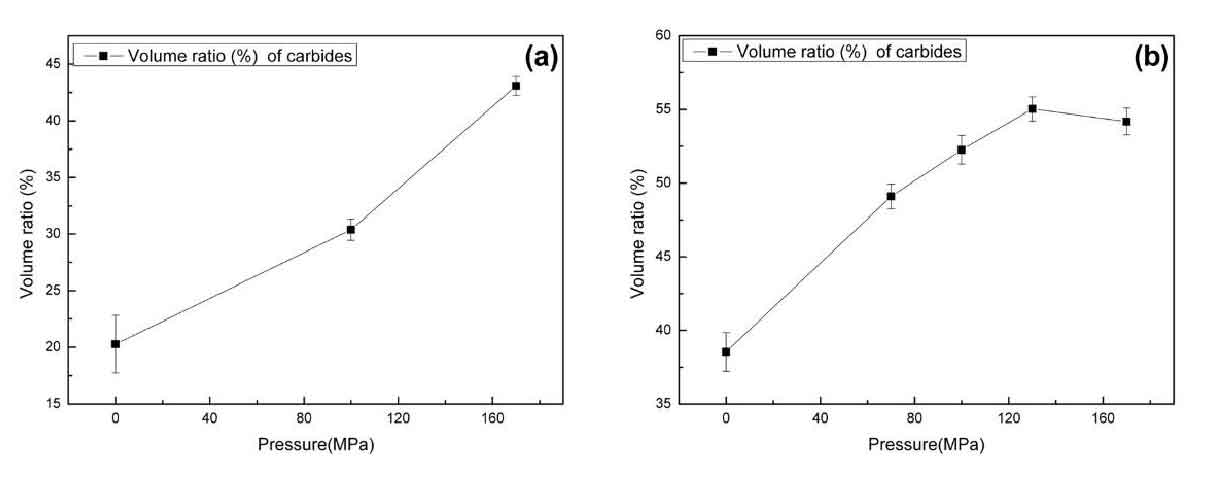# Effect of solidification pressure on total carbide content of chromium white cast iron

In the solidification process of Hypoeutectic chromium white cast iron, the primary phase is austenite, the carbides in the solidification structure are eutectic transformation products, and all eutectic chromium white cast iron are eutectic carbides. Besides eutectic carbides, primary carbides are also included in hypereutectic alloys. The amount of carbides determines the hardness of the alloy, and more carbides leads to higher hardness. However, too much carbides will lead to poor toughness of the alloy, which is not conducive to the wear resistance of the alloy.

By using the image pro plus 6.0 software image analysis software to analyze and count the metallographic picture under 200 times magnification, the change rule of carbide content with pressure is obtained, as shown in Figure 1. With the increase of pressure, the proportion of carbides in the solidification structure increases. In Fig. 1 (a), when the pressure is 170 MPa, the volume ratio of carbide in hypoeutectic alloy 1 # is 2 times of that under normal pressure solidification condition, and the increasing rate basically presents a linear change, and the increasing range is large. Because all the carbides in alloy 1 are eutectic carbides, it can be said that pressure promotes the increase of carbide content in eutectic structure. Combined with the size and quantity of eutectic carbides in metallographic structure picture, it is concluded that pressure can promote the nucleation of eutectic carbides. In Fig. 1 (b), the content of carbide in alloy 2 increases about 1.5 times when the pressure is 130 MPa. With the increase of pressure, the increase of total carbide content changes from linear to nonlinear, and the increase rate gradually slows down. When the pressure is less than 100MPa, the increase range is larger than 100MPa. When the pressure is 160MPa, the total amount of carbide even decreases slightly. In addition, the carbides in alloy 2 consist of primary carbides and eutectic carbides, and the effect of pressure on different carbides content needs to be further explored.Carbide content in alloy under different pressure conditions: (a) Alloy 1#; (b) Alloy 2#

In order to reduce the error of carbide content statistics, the carbon equivalent (CEQ) of different samples should be calculated by the formula

The composition phases of hypoeutectic white cast iron are pearlite, carbide and ledeburite. The relative amount of carbide can be calculated by lever law, and the content of carbide is 11.7 ~ 12.7%.

For hypereutectic white cast iron, the content of carbide can be calculated by formula

For different carbon equivalent samples, the deviation of carbide content is ± 1%, which is relatively small and will not have a substantial impact on the final carbide content.

The amount of carbides in solidification structure of chromium white cast iron depends on the content of carbon and chromium. According to the empirical formula of carbide volume, as shown in the formula, when the Cr content is 11 ~ 26 wt.%, the amount of carbide in alloy 2 can be calculated to be about 38 ~ 39%. As the Cr content in alloy 1 is low, it is not calculated here.